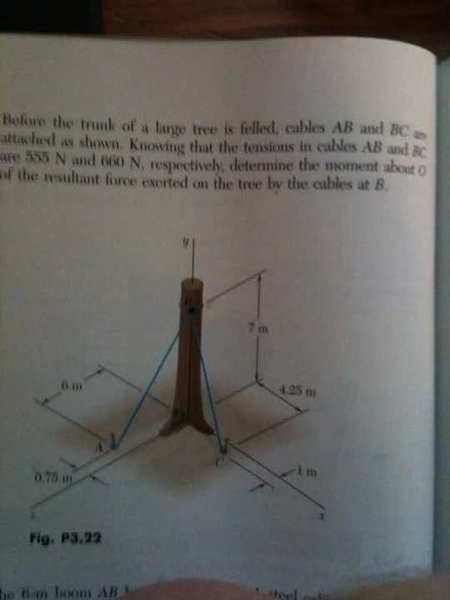# Find the Moment (Torque) of the resultant in 3-D (Statics)

fordrew
Hey guys... This problem is driving me bonkers. I really am not asking you to calculate anything but I only ask how to figure out how to get r to use τ = r x F . My professor did not even teach us anything about resultants and 3-d moments :grumpy:

## Homework StatementMO= τ = r X F

## The Attempt at a Solution

Well, I found that the resultant F = 288.55 i + 985.97 j + 437 k .
The resultant is CORRECT. I can not figure out how to get the distance of this vector in respect to x, y , z for r so I can use the cross product to figure out the moment.

...Or perhaps I have approached the problem all wrong? Is there a different way to solve the problem? ( please do not use any numbers just to make it easier on you)

The book makes no mention of how to solve this problem and it is so so frustrating because even the professor has not done any problems pertaining to this question.

Last edited:

Mentor
Hey guys... This problem is driving me bonkers. I really am not asking you to calculate anything but I only ask how to figure out how to get r to use τ = r x F . My professor did not even teach us anything about resultants and 3-d moments :grumpy:

## Homework StatementMO= τ = r X F

## The Attempt at a Solution

Well, I found that the resultant F = 288.55 i + 985.97 j + 437 k .
The resultant is CORRECT. I can not figure out how to get the distance of this vector in respect to x, y , z for r so I can use the cross product to figure out the moment.

...Or perhaps I have approached the problem all wrong? Is there a different way to solve the problem? ( please do not use any numbers just to make it easier on you)

The book makes no mention of how to solve this problem and it is so so frustrating because even the professor has not done any problems pertaining to this question.

If you are to find the torque about the origin, then the r vector would just be from the origin to the point that the force is applied (where the ropes are attached). It's a little hard to read the picture, but that would be my impression.

fordrew
So the r vector will just be 0i + 7j + 0k? I will test it out right now if it works... the answer is in the back of the book.. So I will get back with the results in a sec

fordrew
well, the answer to the problem is:

3080 i - 2070 k

- 3059 i - 2019 k

maybe my resultant vector is slightly off? And cross product makes i negative =-\

Mentor
well, the answer to the problem is:

3080 i - 2070 k

- 3059 i - 2019 k

maybe my resultant vector is slightly off? And cross product makes i negative =-\

Can you show us your cross product math?

mbram168
Hey guys... This problem is driving me bonkers. I really am not asking you to calculate anything but I only ask how to figure out how to get r to use τ = r x F . My professor did not even teach us anything about resultants and 3-d moments :grumpy:

## Homework StatementMO= τ = r X F

## The Attempt at a Solution

Well, I found that the resultant F = 288.55 i + 985.97 j + 437 k .
The resultant is CORRECT. I can not figure out how to get the distance of this vector in respect to x, y , z for r so I can use the cross product to figure out the moment.

...Or perhaps I have approached the problem all wrong? Is there a different way to solve the problem? ( please do not use any numbers just to make it easier on you)

The book makes no mention of how to solve this problem and it is so so frustrating because even the professor has not done any problems pertaining to this question.

XX Take your moment about point O caused by one of the forces and sum it with the moment about point O caused by the other forces